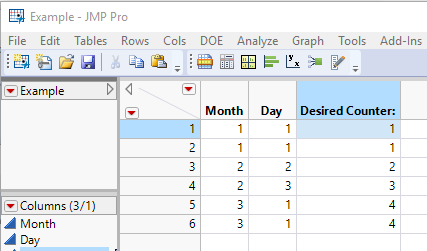Choose Language Hide Translation Bar
Highlighted

## Want to count up when change occurs in either of two data columns

So I have 2 columns of data that have many identical values but which increment at certain points. They are the month and date of data points. Example:

 Month Day Desired Counter: 1 1 1 1 1 1 2 2 2 2 3 3 3 1 4 3 1 4

Ive tried using lag unsuccessfully as I dont want an increment unless a value changes. I've tried different solutions including:

``````If(
Row() == 1, 1,
Lag( :Month, 1 ) & Lag( :Day, 1 ), Lag( :DesiredCounter, 1 )
)``````

A similar solution was posted but didnt work for me as I need to keep both columns into account. Can someone help with this? Thank you

1 ACCEPTED SOLUTION

Accepted Solutions
Highlighted

## Re: Want to count up when change occurs in either of two data columns

Here is a script that creates your sample data table, and applies the formula to the Desired Counter: column

``````New Table( "Example",
New Column( "Month",
Numeric,
"Continuous",
Format( "Best", 12 ),
Set Values( [1, 1, 2, 2, 3, 3] )
),
New Column( "Day",
Numeric,
"Continuous",
Format( "Best", 12 ),
Set Values( [1, 1, 2, 3, 1, 1] )
),
New Column( "Desired Counter:",
Numeric,
"Continuous",
Format( "Best", 12 ),
Formula(
If( Row() == 1,
x = 1,
If( Lag( :Month ) != :Month | Lag( :Day ) != :Day,
x = x + 1
)
);
x;
)
)
)``````

It produces this data table:Jim
4 REPLIES 4
Highlighted

## Re: Want to count up when change occurs in either of two data columns

Here is how I typically do this:

``````If( Row() == 1,
x = 1,
If( Lag( :Month ) != :Month | Lag( :day ) != :Day,
x = x + 1
)
);
x;``````
Jim
Highlighted

## Re: Want to count up when change occurs in either of two data columns

Hey Jim,

Thanks for your reply. So I tried this and I'm just getting a bunch of 1's still. Is there anything else I might need to add? X is our desired counting column right? Thank you

Highlighted

## Re: Want to count up when change occurs in either of two data columns

Here is a script that creates your sample data table, and applies the formula to the Desired Counter: column

``````New Table( "Example",
New Column( "Month",
Numeric,
"Continuous",
Format( "Best", 12 ),
Set Values( [1, 1, 2, 2, 3, 3] )
),
New Column( "Day",
Numeric,
"Continuous",
Format( "Best", 12 ),
Set Values( [1, 1, 2, 3, 1, 1] )
),
New Column( "Desired Counter:",
Numeric,
"Continuous",
Format( "Best", 12 ),
Formula(
If( Row() == 1,
x = 1,
If( Lag( :Month ) != :Month | Lag( :Day ) != :Day,
x = x + 1
)
);
x;
)
)
)``````

It produces this data table:Jim
Highlighted

## Re: Want to count up when change occurs in either of two data columns

Oh, Im sorry, I thought I had to sub a column in for x. Nevermind, it works, thank you!!!

Article Labels

There are no labels assigned to this post.